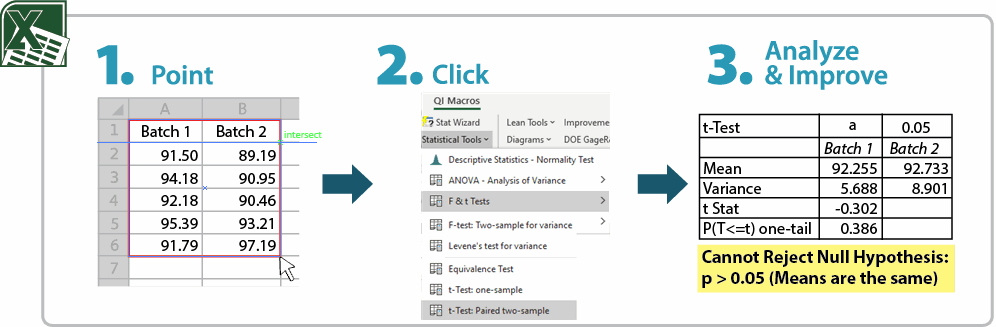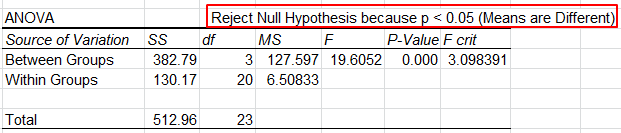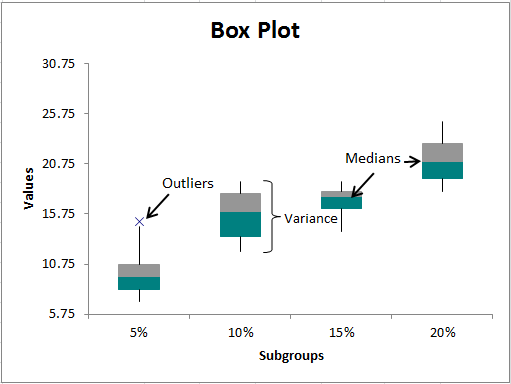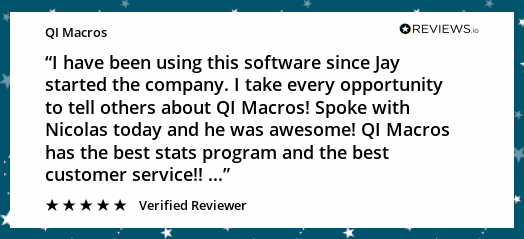# Struggling with Hypothesis Testing in Excel?

## QI Macros Makes Hypothesis Testing Easy, Even If You Don't Know ANYTHING About Hypothesis Testing!

### Go Deeper: What is a Hypothesis Test?

Hypothesis testing helps identify ways to reduce costs and improve quality. Hypothesis testing asks the question: Are two or more sets of data the same or different, statistically.

For companies working to improve operations, hypothesis tests help identify differences between machines, formulas, raw materials, etc. and whether the differences are statistically significant or not. Without such testing, teams can run around changing machine settings, formulas and so on causing more variation. These knee-jerk responses can amplify variation and cause more problems than doing nothing at all.

### Three Types of Hypothesis Tests

• Classical Method - comparing a test statistic to a critical value
• p Value Method - the probability of a test statistic being contrary to the null hypothesis
• Confidence Interval Method - is the test statistic between or outside of the confidence interval

### How to Conduct a Hypothesis Test

1. Define the null (H0) and an alternate (Ha) hypothesis.
2. Conduct the test.
3. Using data from the test:
• Calculate the test statistic and the critical value (t-Test, F-test, z-Test, ANOVA, etc.).
• Calculate a p value and compare it to a  significance level (a) or confidence level (1-a).
4. Interpret the results to determine if you "cannot reject null hypothesis (accept null hypothesis)" or "reject the null hypothesis."Which test do I run?
What do these numbers mean?

### QI Macros for Excel Makes Hypothesis Testing as Easy as 1-2-3!1. Just input your data into an Excel spreadsheet and select it.
2. Click on QI Macros menu, Statistical Tools and the test you want to run (t test, f test, z test, ANOVA, etc.).  If you are not sure which test to run, QI Macros Stat Wizard will analyze your data and run the possible tests for you.
3. QI Macros performs all of the calculations AND interprets the results for you:Hypothesis Test Compare Result What Does It Mean? Classical Method test statistic > critical value  (i.e. F > F crit) Reject the null hypothesis Means or Variances are Different Means or Variances are not the Same Classical Method test statistic < critical value  (i.e. F < F crit) Cannot Reject the null hypothesis/Accept the null hypothesis Means or Variances are the Same Means or Variances are not Different p value Method p value < a Reject the null hypothesis Means or Variances are Different Means or Variances are not the Same p value Method p value > a Cannot Reject the null hypothesis/Accept the null hypothesis Means or Variances are the Same Means or Variances are not Different

### Why Choose QI Macros Statistical Software for Excel?### Easy to Use

• Works Right in Excel
• Interprets p-values for You
• Accurate No-Worry Results
• Free Training Anytime### Proven and Trusted

• 100,000 Users in 80 Countries
• Celebrating 20th Anniversary
• Five Star CNET Rating - Virus Free### Affordable

• Only \$349 USD
Quantity Discounts Available
• No annual fees
• Free Technical Support